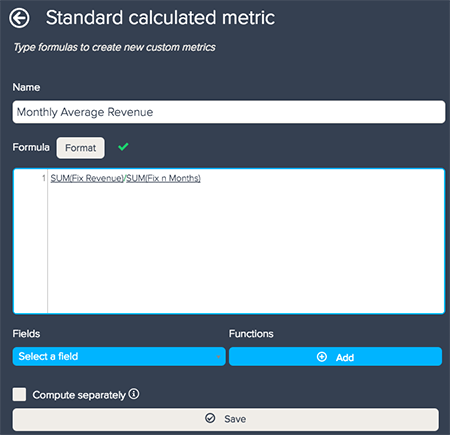Follow

Your standard calculated metrics in BIME are dynamic. They can recalculate based on the attributes used, date periods specified, and filters applied (see Standard calculated metrics). However, if you are comparing across categories or benchmarking, you might want the calculation to remain constant. To achieve this, you can use fixed calculated metrics.

At their simplest, you can use a fixed calculated metric to compare metrics. For example, you might want to compare the average sales amount broken down by a region or a time period against the overall average sales amount.

To create a calculated metric

1. Open the Calculation engine menu in the Query Builder.
2. Select Fixed calculated metric.
3. Enter a name for the metric.
4. Select either the Simple or Advanced option for creating a calculated metric. The Advanced option enables you to type a formula in the same way as a standard calculated metric (see Standard calculated metrics and attributes). The Simple option does not require you to enter any formulas.5. Select a metric from the Will always return the value of this metric drop-down list. For example, to compare the costs by region, select Cost Goods as your metric.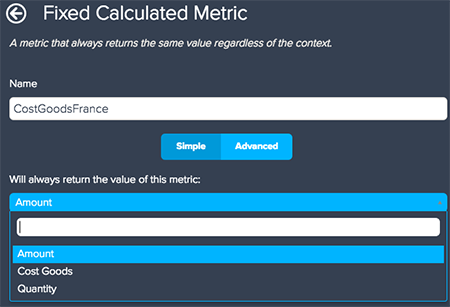6. Check the relevant computing option. There are two available options. You can either use Compute according to the filters in the query to restrict results to your applied filters, or you can check Restricted for the values of an attribute to limit results to a specific attribute's values.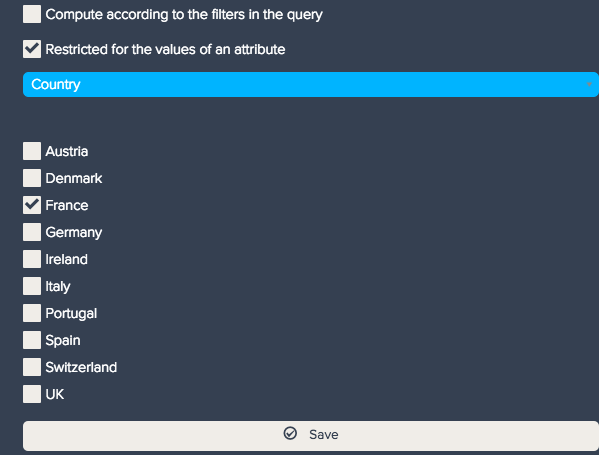7. Click Save when you are finished.
8. Add your calculated metric to Metrics or create a new standard calculated metric.

To compute the average sales amount per attribute, you need to create a standard calculated metric. For example, to calculate the average sales amount per month, use the following calculations:
• A simple fixed calculated metric of the overall Revenue. Select Revenue as your metric and the Compute according to filters in the query option, unless you would like to divide original revenue results by a specific attribute.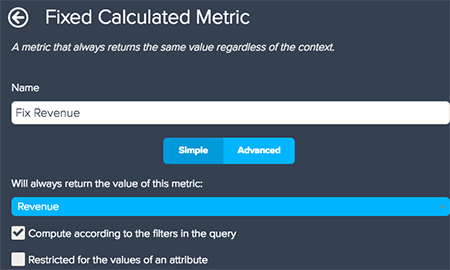• An advanced calculated metric using the DCOUNT_VALUES and your selected attribute.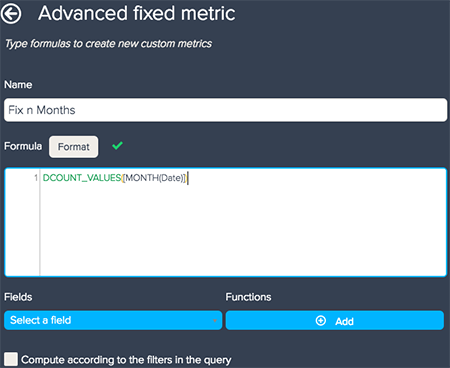• A standard calculated metric dividing the first metric by the second.